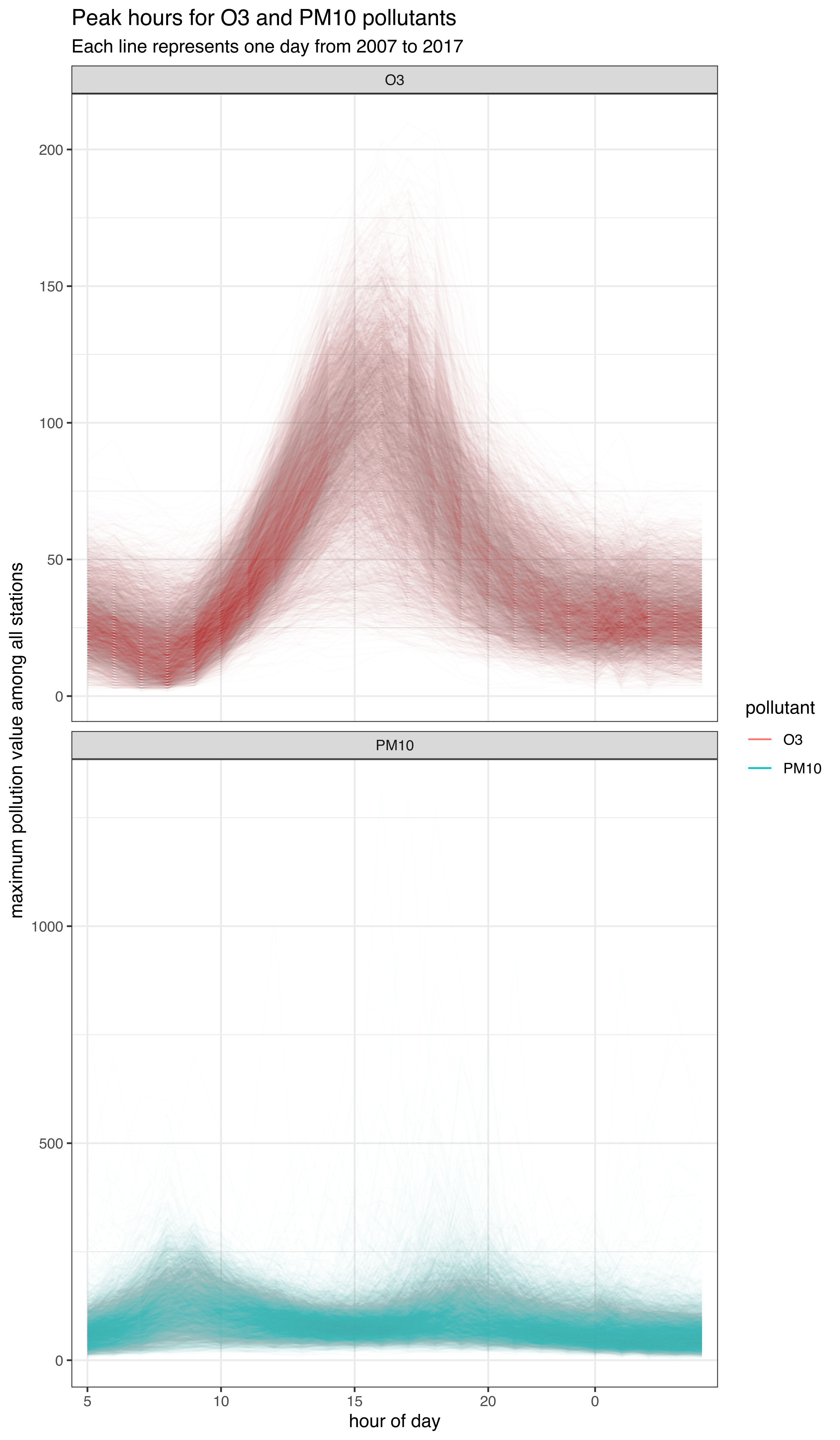Here’s an example of plotting the maximum pollution value for Ozone and PM10, by hour of day. First we load the package necessary for performing the analysis:

## Auto-install required R packages
if (!require("pacman")) install.packages("pacman")
pacman::p_load(aire.zmvm, dplyr, ggplot2, lubridate, stringr)

Once loaded, we can download O3 and PM10M data in the original units using the get_station_data function:

download_horarios <- function(pollutant) {
df <- get_station_data(criterion = "HORARIOS", # Can be MAXIMOS (daily maximum),
# MINIMOS (daily minimum),
# or HORARIOS (hourly average)
pollutant = pollutant, # "SO2", "CO", "NOX", "NO2", "NO", "O3",
# "PM10", "PM25", "WSP", "WDR", "TMP", "RH"
year = 2007:2017) # The earliest year allowed is 1986
df$pollutant <- pollutant # Daily max among all base stations df %>% group_by(date, hour, pollutant) %>% summarise(max = ifelse(all(is.na(value)), NA, base::max(value, na.rm = TRUE))) %>% na.omit() %>% ungroup() } ll_horarios <- mapply(download_horarios, pollutant = c("O3", "PM10"), SIMPLIFY = FALSE) ## summarise() regrouping output by 'date', 'hour' (override with .groups argument) ## summarise() regrouping output by 'date', 'hour' (override with .groups argument) df_horarios <- bind_rows(ll_horarios) ### Dealing with daylight savings time The data returned by get_station_data when the “HORARIOS” criterion is used, includes the date and hour of each measurement. The hour is specified as an offset from midnight and does not include daylight savings time (basically GMT+6). It’s probably a good idea to convert it to local Mexico City time if we want to analyse pollution patterns by hour of day. knitr::kable(head(df_horarios)) date hour pollutant max 2007-01-01 1 O3 11 2007-01-01 2 O3 10 2007-01-01 3 O3 12 2007-01-01 4 O3 13 2007-01-01 5 O3 15 2007-01-01 6 O3 14 # The time is given in hours with no DST # GMT has no DST df_horarios$datetime <- as.POSIXct(
strptime(paste0(df_horarios$date, " ", df_horarios$hour),
"%Y-%m-%d %H", tz = "Etc/GMT+6")
)
# Convert to MXC time
df_horarios$datetime_mxc <- as.POSIXct(format(df_horarios$datetime,
tz="America/Mexico_City",
usetz = TRUE))
df_horarios$hour_dst <- hour(df_horarios$datetime_mxc)

Ozone peaks a couple of hours after midday and PM10 particles peak with commuting hours (according to the Encuesta Origen-Desino 2017), and also sometimes around midnight because of fireworks and trash burning. A phase I contingency is declared when the 24 hour average PM10 concentration exceeds 150 IMECAS in at least two stations and for O3 when the hourly average exceeds 150 IMECAS (the data is shown in the original units of the data, not IMECAS)

df_horarios$hour_dst <- factor(df_horarios$hour_dst,
levels = c(5:23, 0:4),
ordered = TRUE)

ggplot(df_horarios,
aes(hour_dst, max, group = date, color = pollutant)) +
geom_line(alpha = I(1/sqrt((2017-2001)*1565))) +
facet_wrap(~pollutant, scales = "free_y", ncol = 1) +
guides(color = guide_legend("pollutant",
override.aes = list(alpha = 1))) +
theme_bw() +
xlab("hour of day") +
ylab("maximum pollution value among all stations") +
scale_x_discrete(breaks = seq(0, 23, 5)) +
labs(title = "Peak hours for O3 and PM10 pollutants",
subtitle = "Each line represents one day from 2007 to 2017")